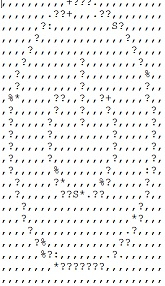Converting an image to ASCII image in Python

PythonServer Side ProgrammingProgramming

In this article we we want to convert a given images into a text bases image also called ASCII image.

Below is the Python program which will take an input imagee and various functions to convert them into grayscale picture and then apply the ASCII characters to create different patterns insert the image. Finally the emails comes text based image this is a series of plane ASCII characters.

Example

from PIL import Image
import os
ASCII_CHARS = [ '#', '?', '%', '.', 'S', '+', '.', '*', ':', ',', '@']
def resize_image(image, new_width=25):
(input__width, input__height) = image.size
aspect_ratio = input__height/float(input__width)
changed_height = int(aspect_ratio * new_width)
changed_image = image.resize((new_width, changed_height))
return changed_image
def make_grey(image):
return image.convert('L')
def pixel_to_ascii(image, range_width=25):
pixels_in_image = list(image.getdata())
pixels_to_chars = [ASCII_CHARS[pixel_value//range_width] for pixel_value in
pixels_in_image]
return "".join(pixels_to_chars)
def image_to_ascii(image, new_width=25):
image = resize_image(image)
image = make_grey(image)
pixels_to_chars = pixel_to_ascii(image)
len_pixels_to_chars = len(pixels_to_chars)
image_ascii = [pixels_to_chars[index: index + new_width] for index in
range(0, len_pixels_to_chars, new_width)]
return "\n".join(image_ascii)
def convert_image(image_filepath):
# image = None
try:
image = Image.open(image_filepath)
except Exception as e:
print("Unable to open image file          {image_filepath}.".format(image_filepath=image_filepath))
print(e)
return
image_ascii = image_to_ascii(image)
f = open(os.path.splitext(image_filepath)+'.txt','w')
f.write(image_ascii)
f.close()
convert_image('D:\\button.jpg')

Output

Running the above code gives us the following result −

Input ImageOutput Image